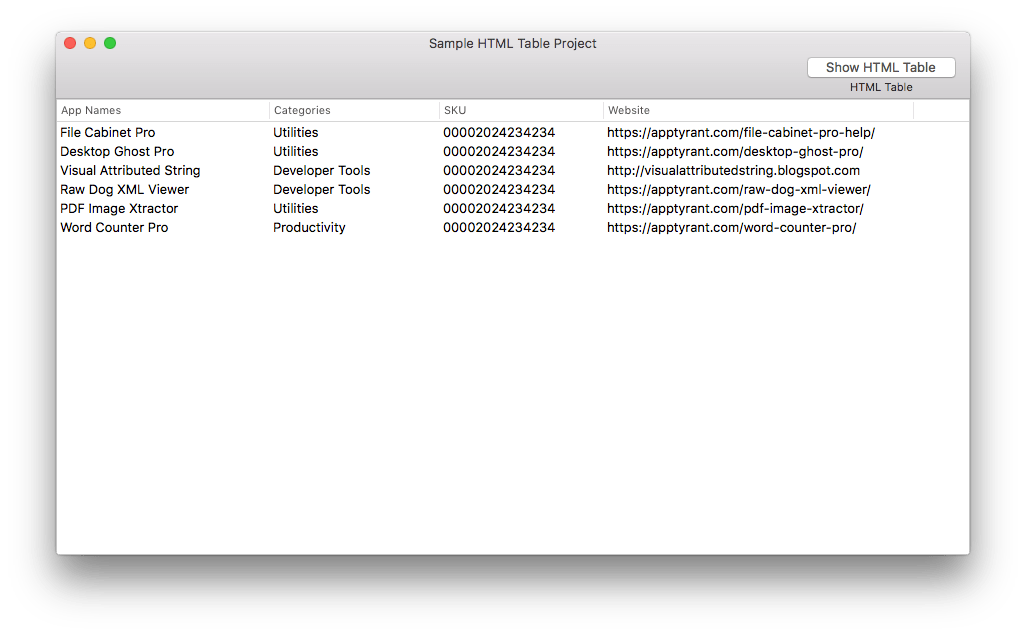## How to Convert Decimal to Hexadecimal

Decimal and hexadecimal are two number systems that are widely used in the fields of computer science and mathematics. Decimal is a base-10 number system that uses the digits 0-9, while hexadecimal is a base-16 number system that uses the digits 0-9 and the letters A-F. In this tutorial, we will show you how to convert decimal to hexadecimal.

### Step 1: Divide the Decimal Number by 16

The first step in converting a decimal number to a hexadecimal number is to divide the decimal number by 16. Write down the quotient and remainder of the division.

For example, let’s convert the decimal number 4096 to hexadecimal.

4096 ÷ 16 = 256
Quotient = 256
Remainder = 0

### Step 2: Convert the Remainder to Hexadecimal

The next step is to convert the remainder from the previous step to hexadecimal. To do this, you can use the following conversion chart:

0 0
1 1
2 2
3 3
4 4
5 5
6 6
7 7
8 8
9 9
10 A
11 B
12 C
13 D
14 E
15 F

In our example, the remainder is 0, so the hexadecimal equivalent is also 0.

### Step 3: Repeat the Process Until the Quotient is 0

The next step is to repeat the previous two steps until the quotient is 0. In our example, we have:

256 ÷ 16 = 16
Quotient = 16
Remainder = 0

16 ÷ 16 = 1
Quotient = 1
Remainder = 0

1 ÷ 16 = 0
Quotient = 0
Remainder = 1

### Step 4: Write the Hexadecimal Equivalent

Once the quotient is 0, you can write down the hexadecimal equivalent by writing the remainders from the last step in reverse order. In our example, the remainders are 0, 0, 0, and 1, so the hexadecimal equivalent of 4096 is 1000.

Therefore, the decimal number 4096 is equivalent to the hexadecimal number 1000.

### Final Thoughts

Converting decimal to hexadecimal may seem complicated at first, but it’s actually quite simple once you understand the process. Just remember to divide the decimal number by 16, convert the remainder to hexadecimal, and repeat the process until the quotient is 0. By following these steps, you can easily convert decimal to hexadecimal for any number.

## Easily Bind an NSProgress Object to an NSProgressIndicator in Objective-C [Open Source]

In UIKit UIProgressView has an observedProgress property. If you set the observedProgress property on a UIProgressView, it will automatically update its appearance when you make changes to the NSProgress object. On macOS (in AppKit at least) NSProgressIndicator does not have an equivalent API. I created a simple category on NSProgressIndicator that adds an observedProgress property on NSProgressIndicator. The source code is available on Github here.

## Adding Force Touch Features to macOS Apps with a Custom Gesture Recognizer [Open Source]

I created a simple subclass of NSGestureRecognizer, ATForceTouchGesture, to simplify the process of adding Force Touch features to macOS apps. You can use this gesture recognizer to add a feature like force clicking to start editing a label (see the screenshot below).There is a sample project available on Github here.

## Exporting NSTableView to HTML [Open Source]

I needed to export the contents of a NSTableView to HTML, so I wrote a little NSTableView subclass in Objective-C to do this.

ATHyperTextTableView is a simple NSTableView subclass that makes exporting a table view to HTML easy. You can customize the look of the exported HTML table with your own CSS too.

Screenshots Below:There is a sample project available on Github here.

## How to Disable NSScrollView Scrolling

Unlike UIScrollView on iOS, NSScrollView on Mac does not have a handy scrollEnabled property for you to set to NO if you need to temporarily disable scrolling in your app.

If you Google around, you can find several posts on websites like stackoverflow that ask questions like How can I disable the vertical scrolling of a NSScrollView? Some have suggested setting the hasVerticalScroller property to NO as the answer. However, the hasVerticalScroller property only effects the visibility of the scroller, a value of NO does not actually prevent scrolling.

There are also ways to constrain scrolling from an NSView subclass, as described in Apple’s documentation here. Constraining scrolling from a view subclass can be useful, but what if you want to just temporarily disable scrolling?

The easiest way to disable scrolling it seems is to subclass NSScrollView and add a BOOL property with a name like scrollingEnabled. Then you can simply override the designated initializers (initWithFrame: and initWithCoder:) and set the ivar of the property to YES as the default value:

```-(instancetype)initWithFrame:(NSRect)frameRect { self = [super initWithFrame:frameRect]; if (self) { [self setUpOnInit]; } return self; }   - (instancetype)initWithCoder:(NSCoder *)coder { self = [super initWithCoder:coder]; if (self) { [self setUpOnInit]; } return self; }   -(void)setUpOnInit { //Set all default values. _scrollingEnabled = YES; }```

Now you can just override the scrollWheel: method and check the property:

```-(void)scrollWheel:(NSEvent *)theEvent { if (self.scrollingEnabled) { [super scrollWheel:theEvent]; } else { //scrolling is disabled. } }```

This technique will work in most cases, but there may be times when a view inside of a scroll view implements autoscrolling behavior (perhaps if the view is a dragging destination). If you need to temporarily disable scrolling in such a case, you should also subclass NSClipView and block scrolling by overriding the -constrainBoundsRect: method like this:

```-(NSRect)constrainBoundsRect:(NSRect)proposedBounds { MyScrollViewSubclass *mainScrollView = (MyScrollViewSubclass*)self.superview;   if (mainScrollView.scrollingEnabled) { return [super constrainBoundsRect:proposedBounds]; } else { //Disabled return self.documentVisibleRect; } }```